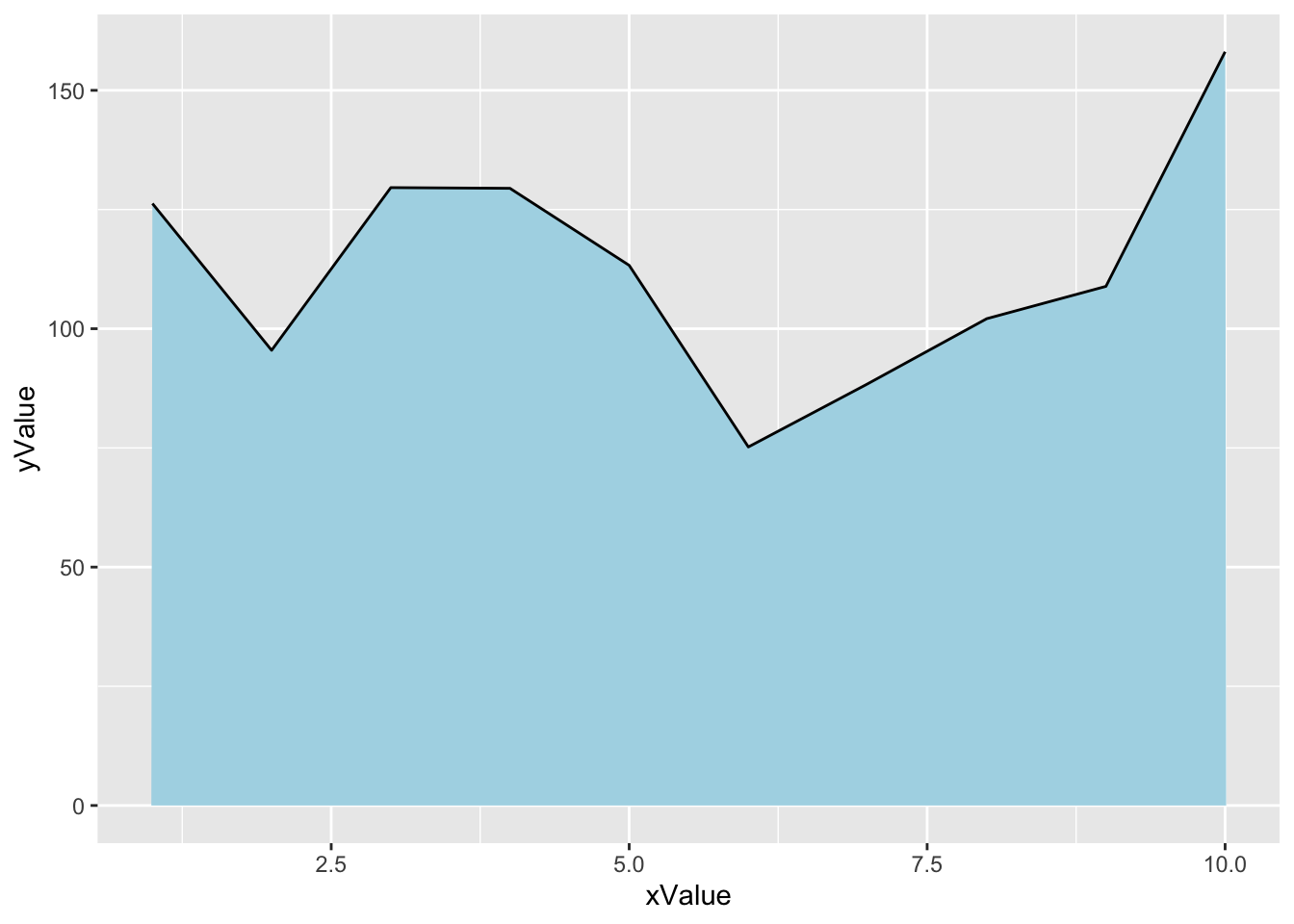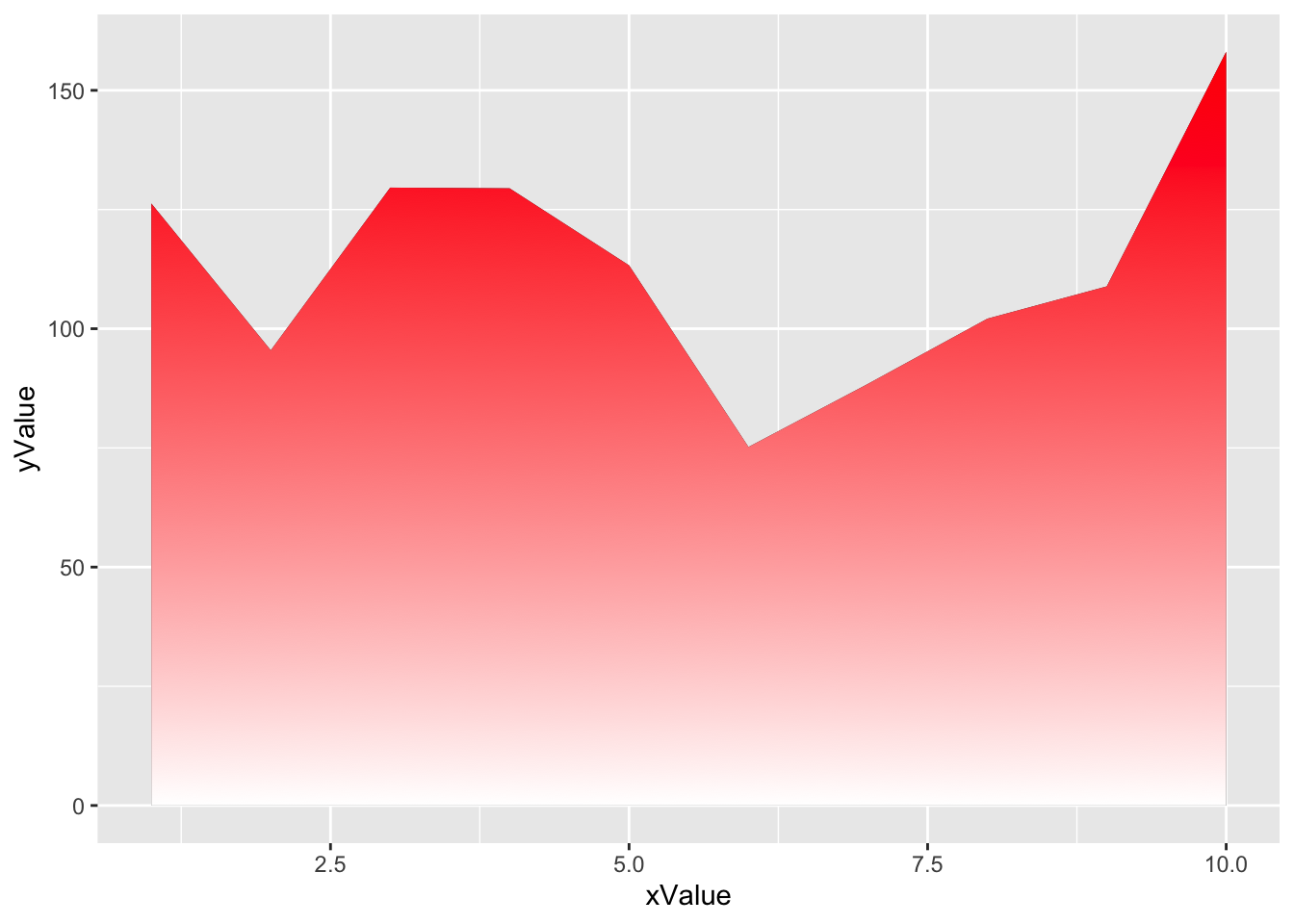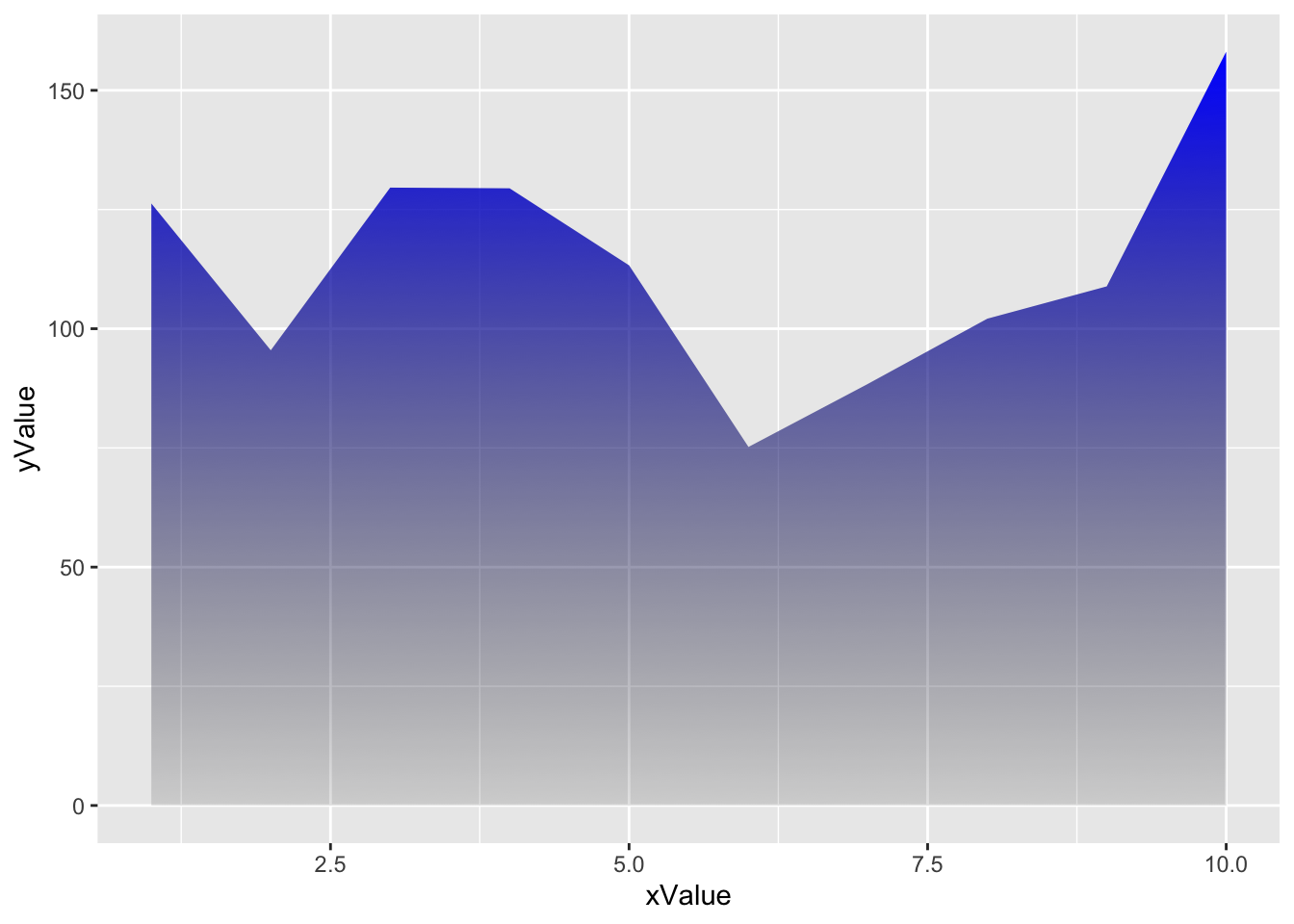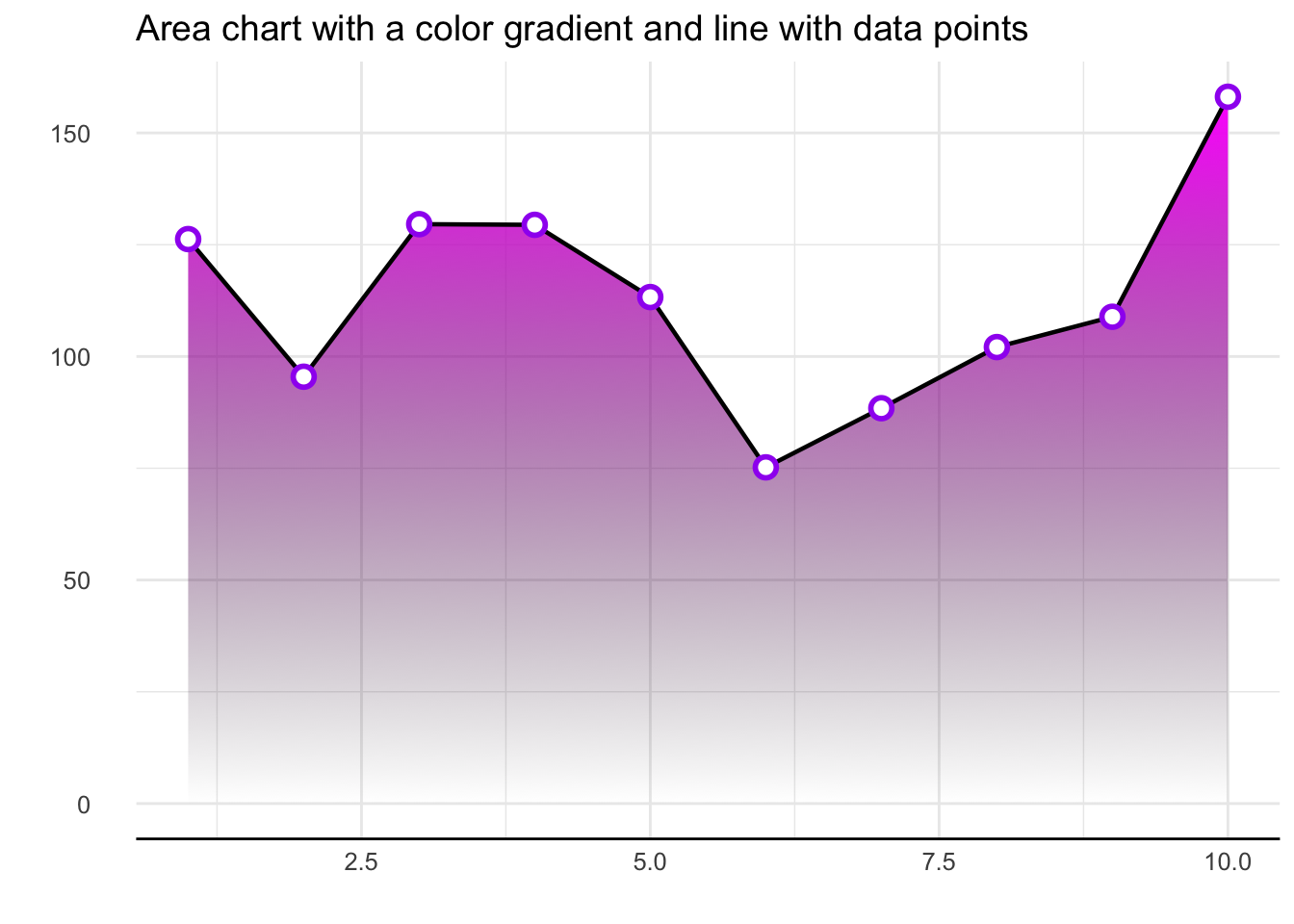This post explains how to create an `area chart` with a color gradient between the bottom line and the line chart.
We’ll go through several examples with reproducible R code using ggplot2 and ggpattern.

# Packages

For this post, we need to load the following libraries:

``````library(ggplot2)
library(ggpattern)
library(hrbrthemes)``````

# Dataset

We create a simple dataset with 2 columns, randomly generated thanks to `runif()` and `rnorm()` functions.

``````set.seed(0)
n = 10
x = 1:n
y = x + rnorm(n=n, mean=100, sd=20)
df = data.frame(xValue = x,
yValue = y)``````

# Most basic area chart

To create an area chart with R, all we need to do is add the `geom_area()` function from ggplot2.

``````ggplot(df, aes(x=xValue, y=yValue)) +
geom_area(colour='black', fill='lightblue')``````To add a color gradient in place of the light blue, we need to use the ggpattern library and define which colors will be used for the graph.

The bottom color is `white` and the top color is `red`.

``````ggplot(df, aes(xValue, yValue)) +
geom_area_pattern(data = df,
pattern_fill = "white",
pattern_fill2 = "red")``````To add transparency to the graphic, simply use colors that are naturally transparent. For example, here we change white to transparent grey.

The bottom color is a transpaent `light grey` (#00000010) and the top color is `blue`.

``````ggplot(df, aes(xValue, yValue)) +
geom_area_pattern(data = df,
fill = "#00000010",
pattern_fill  = "#00000010",
pattern_fill2 = "blue")``````# Fully customized area chart wiht gradient

If we take what we’ve seen above and add other features like changing the `theme`, removing axis lines etc., you end up with an even more beautiful graph.

In this case, we: - add a black line for the upper part of the area chart - change the theme with the hrbrthemes library - remove axis lines

``````ggplot(df, aes(xValue, yValue)) +
geom_area_pattern(data = df,
fill = "#00000000",
pattern_fill  = "#00000000",
pattern_fill2 = "magenta") +
geom_line(data = df, colour = "black", linewidth = 0.8) +
geom_point(shape = 16, size = 4.5, colour = "purple") +
geom_point(shape = 16, size = 2.5, colour = "white") +
ggtitle("Area chart with a color gradient and line with data points") +
theme_bw() +
theme(plot.title = element_text(size = 14),
panel.border       = element_blank(),
axis.line.x        = element_line(),
text               = element_text(size = 12),
axis.ticks         = element_blank(),
axis.text.y        = element_text(margin = margin(0,15,0,0, unit = "pt"))) +
scale_alpha_identity() + labs(x="",y="")``````# Conclusion

This post explained how to add a color gradient to an area chart using the ggpattern library. For more area chart examples, see the dedicated section.

Related chart types

## Contact

This document is a work by Yan Holtz. Any feedback is highly encouraged. You can fill an issue on Github, drop me a message on Twitter, or send an email pasting yan.holtz.data with gmail.com.